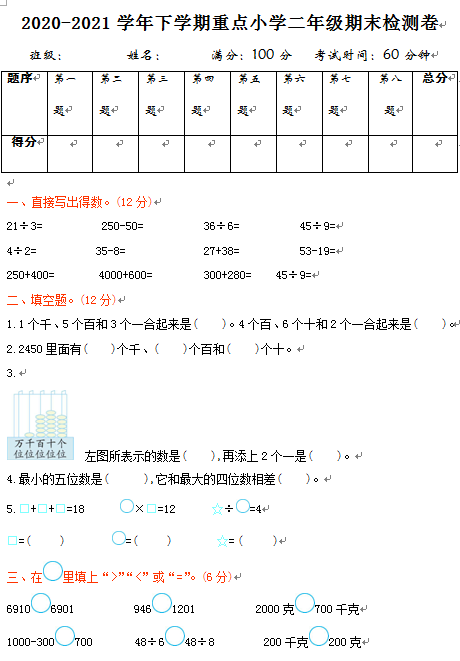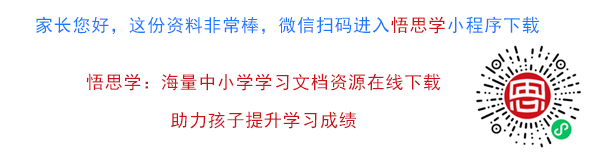2020-2021学年下学期重点小学二年级期末检测卷

21÷3=　　　　　250-50=　　　　　36÷6=　　　　　45÷9=

4÷2=            35-8=              27+38=             53-19=

250+400=          4000+600=            300+280=     45÷9=

【DOC文档6页】2020-2021学年下学期重点小学二年级数学期末检测卷1（含参考答案）A4电子版资料_可直接打印_会员免费下载##### 评论信息